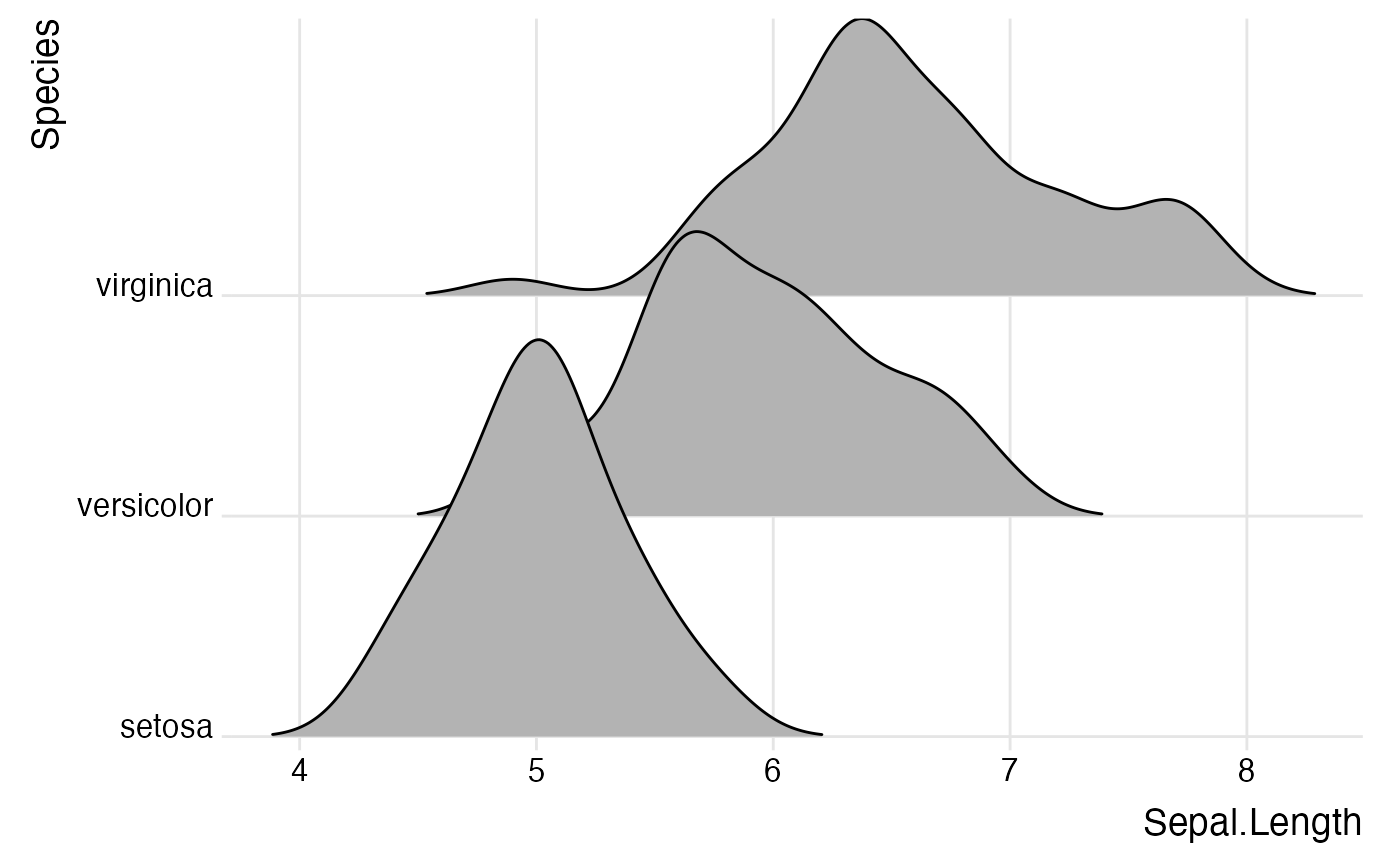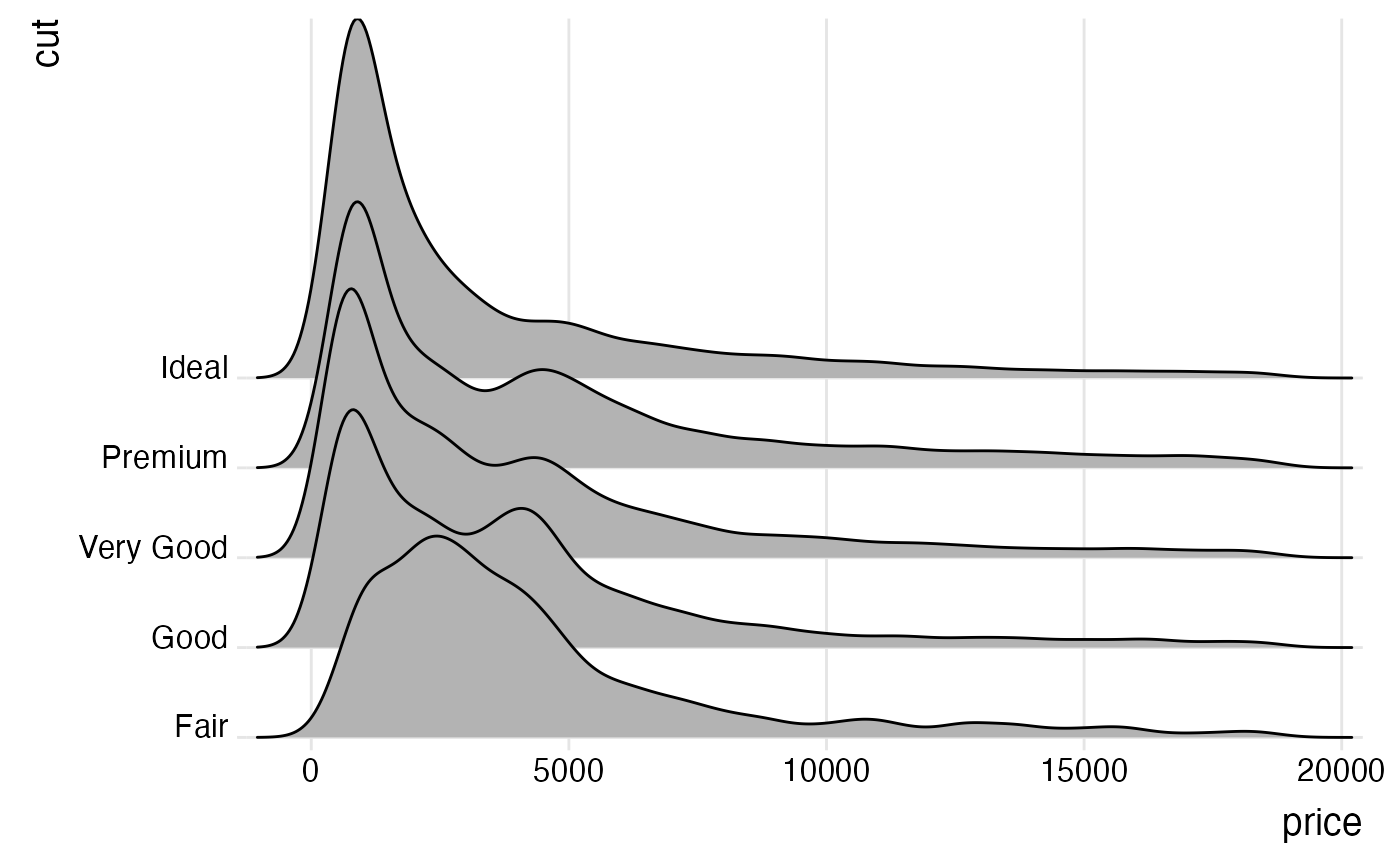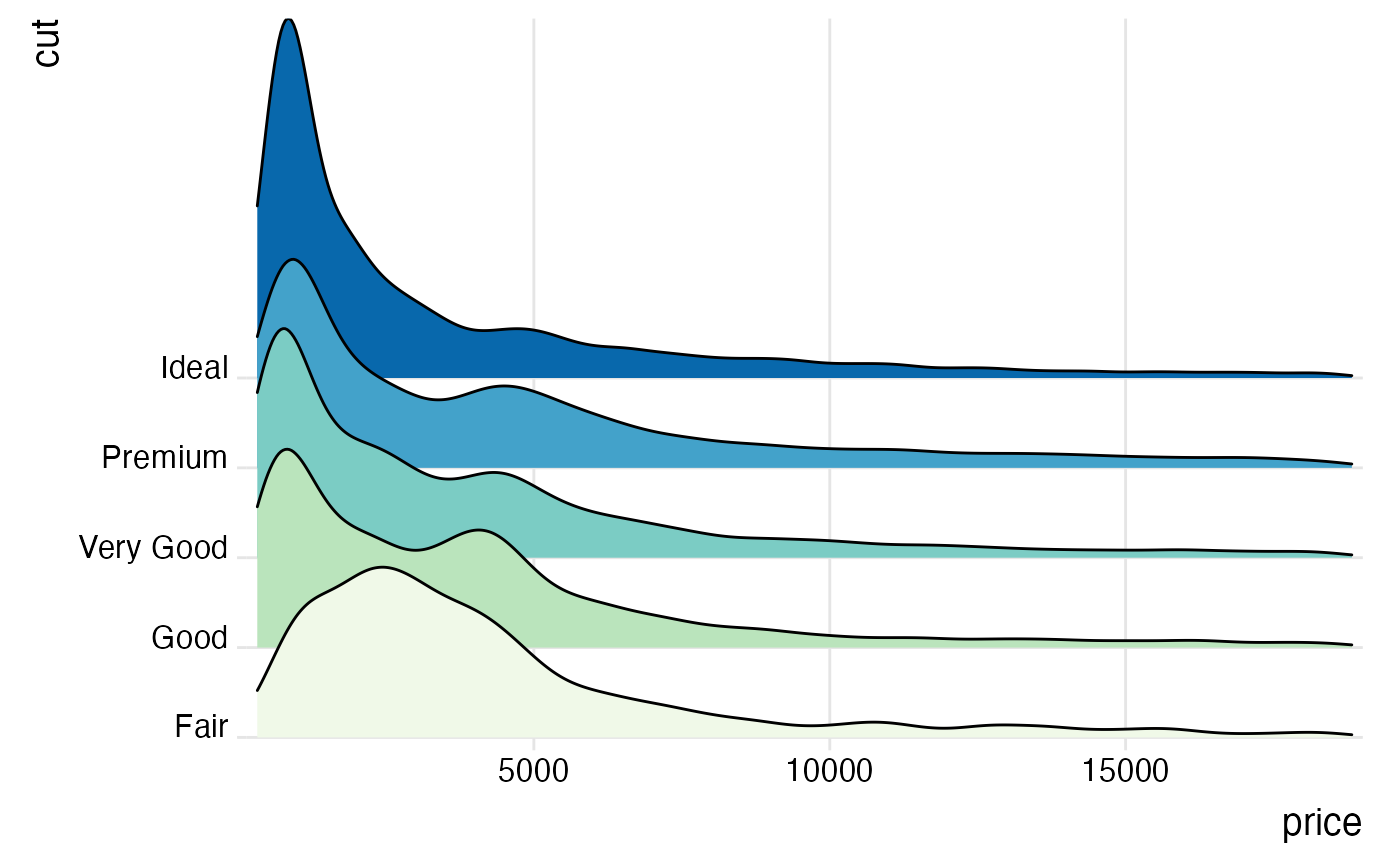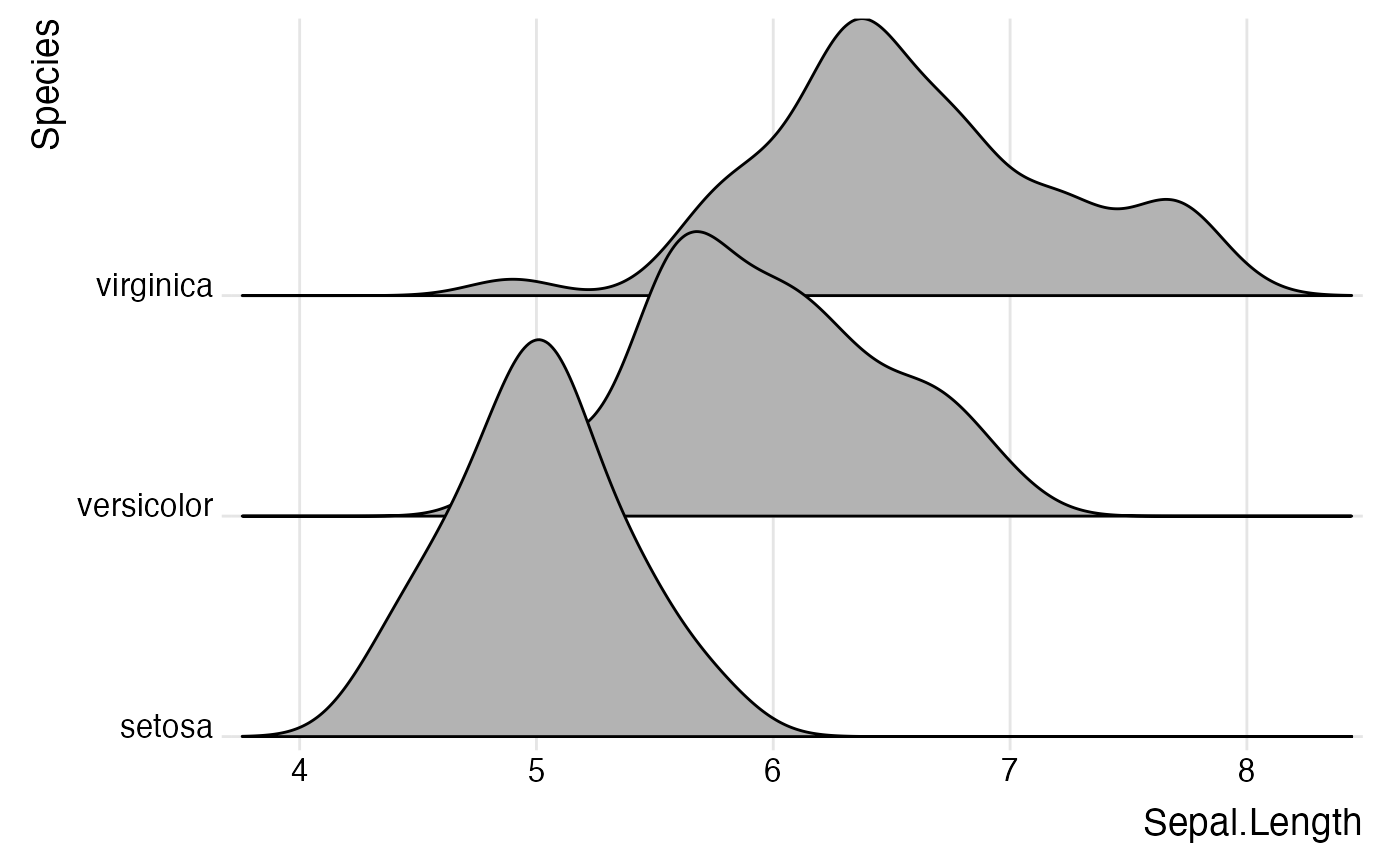geom_density_ridges arranges multiple density plots in a staggered fashion, as in the cover of the famous Joy Division album Unknown Pleasures.

geom_density_ridges(
mapping = NULL,
data = NULL,
stat = "density_ridges",
position = "points_sina",
panel_scaling = TRUE,
na.rm = FALSE,
show.legend = NA,
inherit.aes = TRUE,
...
)

geom_density_ridges2(
mapping = NULL,
data = NULL,
stat = "density_ridges",
position = "points_sina",
panel_scaling = TRUE,
na.rm = FALSE,
show.legend = NA,
inherit.aes = TRUE,
...
)

## Arguments

mapping

Set of aesthetic mappings created by aes() or aes_(). If specified and inherit.aes = TRUE (the default), it is combined with the default mapping at the top level of the plot. You must supply mapping if there is no plot mapping.

data

The data to be displayed in this layer. There are three options:

If NULL, the default, the data is inherited from the plot data as specified in the call to ggplot().

A data.frame, or other object, will override the plot data.

A function will be called with a single argument, the plot data. The return value must be a data.frame., and will be used as the layer data.

stat

The statistical transformation to use on the data for this layer, as a string.

position

Position adjustment, either as a string, or the result of a call to a position adjustment function.

panel_scaling

If TRUE, the default, relative scaling is calculated separately for each panel. If FALSE, relative scaling is calculated globally.

na.rm

If FALSE, the default, missing values are removed with a warning. If TRUE, missing values are silently removed.

show.legend

logical. Should this layer be included in the legends? NA, the default, includes if any aesthetics are mapped. FALSE never includes, and TRUE always includes.

inherit.aes

If FALSE, overrides the default aesthetics, rather than combining with them.

...

other arguments passed on to layer(). These are often aesthetics, used to set an aesthetic to a fixed value, like color = "red" or size = 3. They may also be parameters to the paired geom/stat.

## Details

By default, this geom calculates densities from the point data mapped onto the x axis. If density calculation is not wanted, use stat="identity" or use geom_ridgeline. The difference between geom_density_ridges and geom_ridgeline is that geom_density_ridges will provide automatic scaling of the ridgelines (controlled by the scale aesthetic), whereas geom_ridgeline will plot the data as is. Note that when you set stat="identity", the height aesthetic must be provided.

Note that the default stat_density_ridges makes joint density estimation across all datasets. This may not generate the desired result when using faceted plots. As an alternative, you can set stat = "density" to use stat_density. In this case, it is required to add the aesthetic mapping height = ..density.. (see examples).

## Aesthetics

Required aesthetics are in bold.

• x

• y

• group Defines the grouping. Not needed if a categorical variable is mapped onto y, but needed otherwise. Will typically be the same variable as is mapped to y.

• height The height of each ridgeline at the respective x value. Automatically calculated and provided by stat_density_ridges if the default stat is not changed.

• scale A scaling factor to scale the height of the ridgelines relative to the spacing between them. A value of 1 indicates that the maximum point of any ridgeline touches the baseline right above, assuming even spacing between baselines.

• rel_min_height Lines with heights below this cutoff will be removed. The cutoff is measured relative to the overall maximum, so rel_min_height=0.01 would remove everything that is 1\ ridgelines. Default is 0, so nothing is removed. alpha

• colour, fill, group, alpha, linetype, size, as in geom_ridgeline.

• point_shape, point_colour, point_size, point_fill, point_alpha, point_stroke, as in geom_ridgeline.

## Examples

library(ggplot2)

# set the rel_min_height argument to remove tails
ggplot(iris, aes(x = Sepal.Length, y = Species)) +
geom_density_ridges(rel_min_height = 0.005) +
scale_y_discrete(expand = c(0.01, 0)) +
scale_x_continuous(expand = c(0.01, 0)) +
theme_ridges()
#> Picking joint bandwidth of 0.181# set the scale to determine how much overlap there is among the plots
ggplot(diamonds, aes(x = price, y = cut)) +
geom_density_ridges(scale = 4) +
scale_y_discrete(expand = c(0.01, 0)) +
scale_x_continuous(expand = c(0.01, 0)) +
theme_ridges()
#> Picking joint bandwidth of 458# the same figure with colors, and using the ggplot2 density stat
ggplot(diamonds, aes(x = price, y = cut, fill = cut, height = ..density..)) +
geom_density_ridges(scale = 4, stat = "density") +
scale_y_discrete(expand = c(0.01, 0)) +
scale_x_continuous(expand = c(0.01, 0)) +
scale_fill_brewer(palette = 4) +
theme_ridges() + theme(legend.position = "none")# use geom_density_ridges2() instead of geom_density_ridges() for solid polygons
ggplot(iris, aes(x = Sepal.Length, y = Species)) +
geom_density_ridges2() +
scale_y_discrete(expand = c(0.01, 0)) +
scale_x_continuous(expand = c(0.01, 0)) +
theme_ridges()
#> Picking joint bandwidth of 0.181